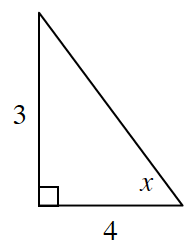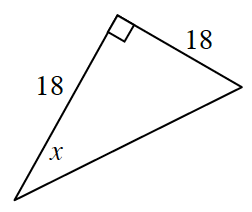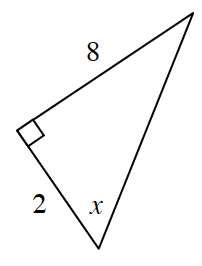### Home > INT2 > Chapter 2 > Lesson 2.3.1 > Problem2-88

2-88.

Based on the measurements provided for each triangle below, determine whether the measure of $x$ must be more than, less than, or equal to $45^\circ$.

See problem 4-28 for more help.

1.Think about what the slope ratio must be for the angle to be $45^\circ$.

1.Use the Pythagorean Theorem to solve for the missing leg. What does this length tell you about the angle's measure?

1.Use the same method as in part (a).

More than $45^\circ$a.